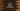# Write a C program to convert an integer value to character## C program to convert an integer to character:

In this post, we will learn different ways to convert an integer to character in C programming language.We have different ways to convert an integer value to character. We will check these methods with examples for each.

### Method 1: Assign an integer value to character value:

We can simply assign an int value to char value to do the conversion. If we have an integer variable, or int variable, we can convert it to a character variable or char variable directly by using this method.

Let’s take a look at the below program:

``````#include <stdio.h>

int main() {
int givenValue = 68;
char resultChar = givenValue;

printf("resultChar: %c",resultChar);

return 0;
}``````

Here,

• givenValue is an integer value and 68 is assigned to it.
• We are assigning its value to resultChar, which is a char variable.
• The printf statement is printing the value of resultChar.

If you run this program, it will print the below output:

``D``

The ASCII value of D is 68. It is converted to the character using the assignment operator.

### Method 2: Convert a digit to character:

We can also convert a digit to character. For example, we can convert 7 to its character value, ‘7’. For that, we need to add ‘0’ with it before doing the conversion.

Adding ‘0’ will add 48 to the digit, which will change the number to the correct ASCII value.

Below program does that:

``````#include <stdio.h>

int main() {
int givenValue = 6;
char resultChar = givenValue + '0';

printf("resultChar: %c",resultChar);

return 0;
}``````

It will print:

``resultChar: 6``

So, we converted int 6 to char ‘6’.